# Volume and capacity – calibrating bottles

Students compare and order several objects based on volume and capacity using appropriate uniform informal units.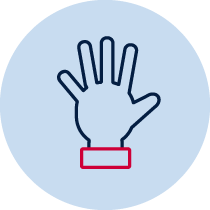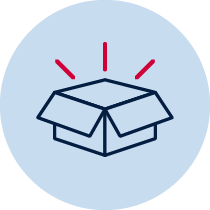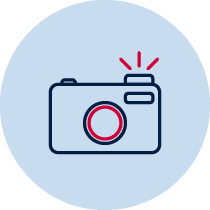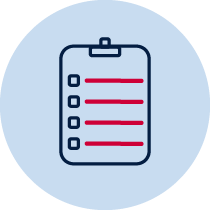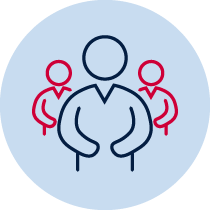Practical Resource required Take photo Teacher observation Collaboration

## Measurement – volume and capacity

Measures, records, compares and estimates volumes and capacities using uniform informal units MA1-11MG

Describes mathematical situations and methods using everyday and some mathematical language, actions, materials, diagrams and symbols MA1-1WM

supports conclusions by explaining or demonstrating how answers were obtained MA1-3WM

## Content

Compare and order several objects based on volume and capacity using appropriate uniform informal units.

## National Numeracy Learning Progression mapping to the NSW mathematics syllabus

When working towards the outcome MA1-11MG the sub-elements (and levels) of understanding units of measurement (UuM3-UuM5) describe observable behaviours that can aid teachers in making evidence-based decisions about student development and future learning.

## Materials

• cup or similar measuring device
• larger container to pour filling material into (for example, soft drink bottle or milk bottle)
• markers
• calibrating bottles (DOCX 70 KB)

## Teacher instructions

The purpose of this task is to gauge students’ understanding of initial volume and capacity concepts such as:

• estimating the capacities using uniform informal units
• recording capacities using uniform informal units
• creating and using a measuring device for capacity that is calibrated in uniform informal units

Ask students, ‘How could we work out how many cups (like this) the large container can hold?’ Students will estimate how many cups they think the large container will hold.

Students will use a cup or similar informal measuring device to calibrate a larger container. The students can complete the task in pairs and record their answers in the spaces provided. The teacher may read the questions to the students if required. Before starting, students are asked to estimate how many cups of water they think it will take to fill the larger container.

Students fill the larger container.

Students discuss actions and results.

Students compare their estimation with the actual result and are asked to record what they noticed.

The teacher can take photos and ask students to describe what they are doing and record observations as they go.

## Student instructions

How many cups of water do you estimate will be needed to fill the larger container?

Think about how you will prove how many cups of water will be needed to fill the larger container. Work with your partner to find out how many cups will fill the larger container and how you will record this.

Write down what you noticed.

Compare your estimate with your result. Write or draw what you notice.

## Possible areas for further exploration

Students will need to explore the language of capacity such as container, full, fill, empty, liquid, solid, measure, estimate, gap.

Students need experience in filling containers both with continuous material (for example, water) and with discrete objects (for example, marbles). The use of continuous materials leads to measurement using the units litre and millilitre in later stages.

## Where to next?

Calibrating a container using uniform informal units is a precursor to students using measuring cylinders calibrated informal units such as litres and millilitres.

Students could fill two or more different sized containers using the same material, marking the container to calibrate as they go and then compare the capacities.

Students could fill a container with two different materials, such as water and then marbles, and compare the results.

Mathematics K-6 Sample Units of Work p.75